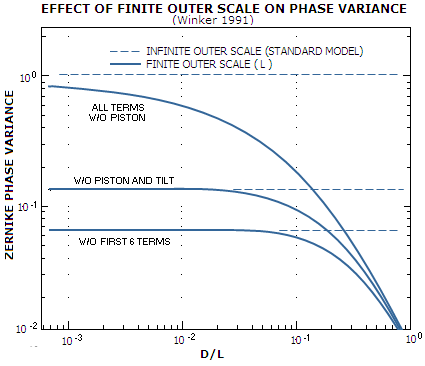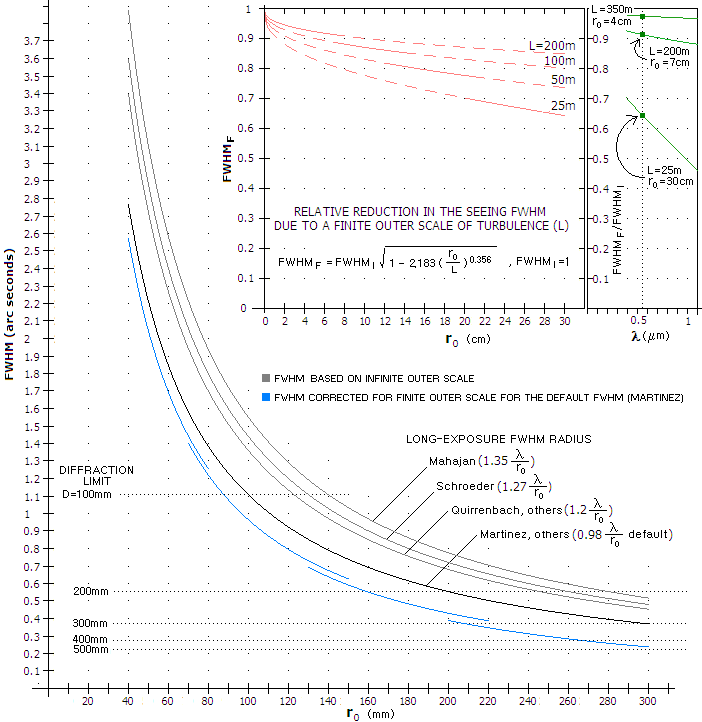telescopeѲptics.net          ▪▪▪▪                                             CONTENTS

# 5.1.2. Limitations to the standard Kolmogorov model of turbulence

The conventional Kolmogorov model of atmospheric turbulence is strictly valid for an inertial range of turbulence with the size much smaller than its outer scale - the large scale air movement at which the turbulence is initiated - and much larger than its inner scale, at which the turbulence is dissipated by air viscosity. However, the effect is calculated by integrating contributions to the refractive index fluctuations - and to the corresponding phase and wave error - on the scale from zero to infinity, assuming an infinite outer scale of turbulence (OST). If the telescope aperture is much smaller than OST, this general approach does not result in a significant error, even if OST is always finite. However, out in the field this is often not the case, and the error in applying this general concept may not be negligible even at quite small apertures. This is particularly the case in good seeing, when - usually - the lower level turbulence dominates, with the OST there possibly smaller than 10m.

Integrating from zero instead from some finite (actual) inner turbulence scale also induces error, but since it is limited to the smallest-scale contributions to wavefront disturbance it is much smaller than the error due to assumed infinite OST, and generally negligible in comparison.In 1991, D.M. Winker investigated the consequences of using a finite OST for calculations of seeing-induced error. As a function of the ratio of the aperture diameter (D) to OST (L), the seeing induced long-exposure error, given as phase variance over Zernike-decomposed  wavefront normalized to (D/r0)5/6, shows significant reduction as the aperture increases relative to the (finite) OST. The effect is the greatest for the first two (tilt) Zernike terms. As the top solid line indicates, with all terms except piston included, not insignificant error reduction of nearly 20% occurs even in an aperture 1,000 times smaller than OST. Even in poor seeing, when the upper level turbulence dominates, and the OST there may reach a few hundred meters, that would be an aperture of up to 12 inches in diameter, or even larger. While the tilt error component may not be fully present, even in poor seeing, in visual observing at these aperture sizes, it is for the better part, and the effect wouldn't be negligible.

In good seeing, when the OST can be expected to be in the 50-100m range, this reduction ratio would, correspondingly, apply to 50-100mm aperture diameter. But at these aperture sizes the tilt component is not a significant error source in visual observing. However, a hundred times smaller aperture is still within the amateurs range. In a 0.5m aperture, with the good seeing r0 of around 10cm, D/r0~5 and the long-exposure phase variance 1.03(D/r0)5/3 being the standard deviation 2πφ squared - φ being the phase analog of the RMS wavefront error - the variance is 3.94 and the corresponding RMS wavefront error is 0.32. At this D/r0 level, most of the tilt component is effectively in the visual seeing error; taking that 2/3 of it is, and knowing that the tilt component makes 64% of the entire (long-exposure) seeing error gives that 40% reduction in the tilt error indicated by the plot for D/L=0.01 results in some 22% smaller RMS wavefront error. Specifically, 0.2 vs. 0.25.

For the long-exposure error, the reduction is from 3.94 to 2.36 in the variance, hence from 0.32 to 0.24 in the RMS (since 2π is a constant, the RMS changes as the square root of the change in variance).

The roughness component of the seeing error, i.e. the error remnant with the tilt removed, is much less sensitive to the use of a finite OST in calculation. The difference becomes significant only at D/L~0.1 and larger, and at even larger D/L values when the first 6 Zernike terms (Noll's scheme) - piston, tilt (horizontal and vertical), defocus and astigmatism (horizontal and vertical) are removed.

The effect of a finite OST on the Kolmogorov-based Zernike wavefront decomposition is a reduction in the error as a whole, reduction in the relative contribution of the image motion component (tilt terms) and increase in the relative contribution of the roughness error component. Specific ratio of motion vs. roughness component depends on the aperture-to-OST (D/L) ratio: larger ratio values result in the relative increase of the roughness component. At D/L~0.001, the error is reduced by about 20% which, with all of it coming from the reduction in tilt (image motion) contribution, increases the relative roughness variance component from 0.134 to about 0.16 (i.e. the roughness RMS wavefront error component from 36% to about 40%). At D/L~0.01 and 40% error reduction, still entirely due to the smaller tilt component, the relative roughness component in the error increases to ~0.22 (~46% of the RMS error), and so on.

Other authors had also addressed this issue. An interesting angle is the effect of finite OST on the seeing FWHM (On the Difference between Seeing and Image Quality: When the Turbulence Outer Scale Enters the Game, Martinez et al. 2010). As shown on the graph below, a finite OST results in a smaller seeing FWHM, the smaller OST, the more so. Similarly to Winker's modeling, limiting the maximum OST size takes out significant portion of large-scale contributions to wavefront disturbances due to turbulence, hence most of the resulting error reduction is in the tip-tilt component, i.e. random motion of image as a whole. The degree of FWHM reduction vs. Kolmogorov (infinite OST) model due to it is approximated (Tokovinin, 2002) based on the von Kàrmàn finite-OST turbulence model.The "default" seeing FWHM for the Kolmogorov model, FWHMI=0.976λ/r0 (used in Martinez et al.) is for λ=500nm and zero zenith angle, is valid under assumption of an aperture infinitely large vs. r0 which, again, is not fulfilled in field conditions (obviously, since it would imply seeing FWHM for D=r0 slightly better than with zero image motion). For large ground telescopes, r0 is not negligibly small and the resolution (theoretical seeing FWHM) is somewhat worse. The most frequently quoted figure is 1.2λ/r0, and the calculation specifically addressing the seeing FWHM in the proximity D=r0 gives long-exposure FWHM as 1.35λ/r0 radians (i.e. 206,265 as many arc seconds).

The graph shows the reduced FWHM (FWHMF, with the subscripts "F" and "I" referring to finite and infinite OST, respectively) vs. "default" Kolmogorov FWHM (blue vs. black), but it is fair to assume that the relative reduction (top left) would be similar for other FWHM modes as well. Since OST size generally increases with turbulence - or, rather, the other way around, as more energy is injected into the air - the reduced FWHM is plotted for four arbitrary, partly overlapping ranges with the averaged OST values from 200m, roughly corresponding to the average ~2 arc second seeing, to 25m, roughly corresponding to 0.5 arc second seeing (more accurate plot would have been the one interpolated through the blue graph segments). Graphs indicate that the nominal FWHM reduction due to finite OST decreases, and its relative reduction increases with better seeing.

The reduction shown on bottom and top left is for r0 values in the visible range of wavelengths (centered at ~550nm). Since r0 varies with the wavelength, as λ1.2, FWHM reduction due to finite OST increases into the infrared, the smaller OST the more so. For instance, if the relative FWHM reduction (top right) is for r0 values for λ~550nm, going to λ~1μm would result in about twice larger r0, and the reduction given by the relation for r0~200mm at λ=550nm (i.e. ~400mm at λ=1μm) and L~25m would have been 0.5, about 40% greater than at 550nm. Top inset at right (green) illustrates the range and dynamics of FWHM reduction in a wider spectral window, from 385 to 1100nm. The reduction is always greater for longer wavelengths. However, it is near negligible in poor seeing (top), quite modest in the average seeing (middle), while becoming significant in excellent seeing (bottom).

Following page addresses in more details how the effect of atmospheric turbulence relates to the aperture size.

5.1.3. Seeing and aperture